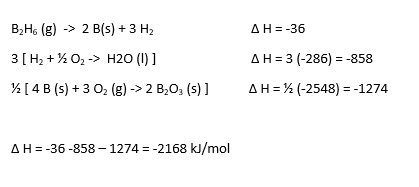# AP Chemistry : Hess' Law

## Example Questions

### Example Question #1 : Hess' Law

Using the following data to calculate the enthalpy change in:  3 NO2(g) + H2O (l) ->  2 HNO3 (aq) +  NO (g)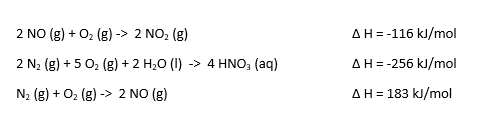-68.5 kJ/mol

137 kJ/mol

-137 kJ/mol

264 kJ/mol

68.5 kJ/mol

-137 kJ/mol

Explanation: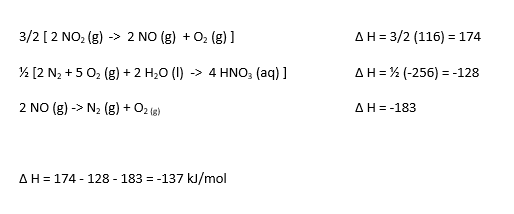### Example Question #2 : Hess' Law

Using the following data to calculate the enthalpy change in:  2 CH4 (g) + 3 O2-> 2 CO (g) + 4 H2O (l)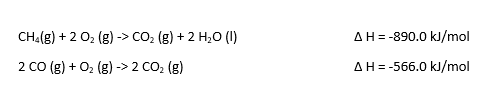214.2 kJ/mol

418.4 kJ/mol

-214.2 kJ/mol

-418.4 kJ/mol

-1214 kJ/mol

-1214 kJ/mol

Explanation: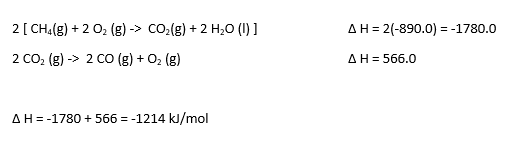### Example Question #3 : Hess' Law

Using the following data to calculate the enthalpy change in:  C4H10O(l)  ->  C4H8 (g)  +  H2O (g)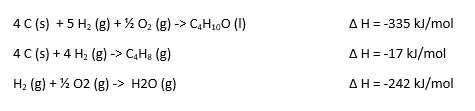-17 kJ/mol

76 kJ/mol

152 kJ/mol

-76 kJ/mol

-152 kJ/mol

76 kJ/mol

Explanation: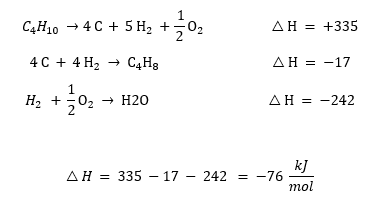### Example Question #4 : Hess' Law

Using the following data to calculate the enthalpy change in:  CaO (s) + H2O (l)  ->  Ca(OH)2 (aq)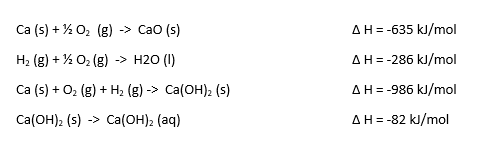-147 kJ/mol

-124 kJ/mol

124 kJ/mol

147 kJ/mol

-62 kJ/mol

-147 kJ/mol

Explanation: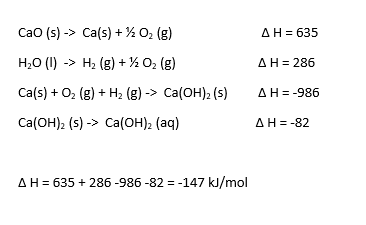### Example Question #5 : Hess' Law

Using the following data to calculate the enthalpy change in:  B2H6 (g) + 3 O2 (g) -> B2O3 (s) + 3 H2O (l)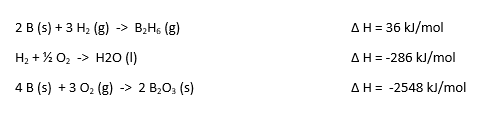-2168 kJ/mol

1084 kJ/mol

3252 kJ/mol

-1084 kJ/mol

2168 kJ/mol